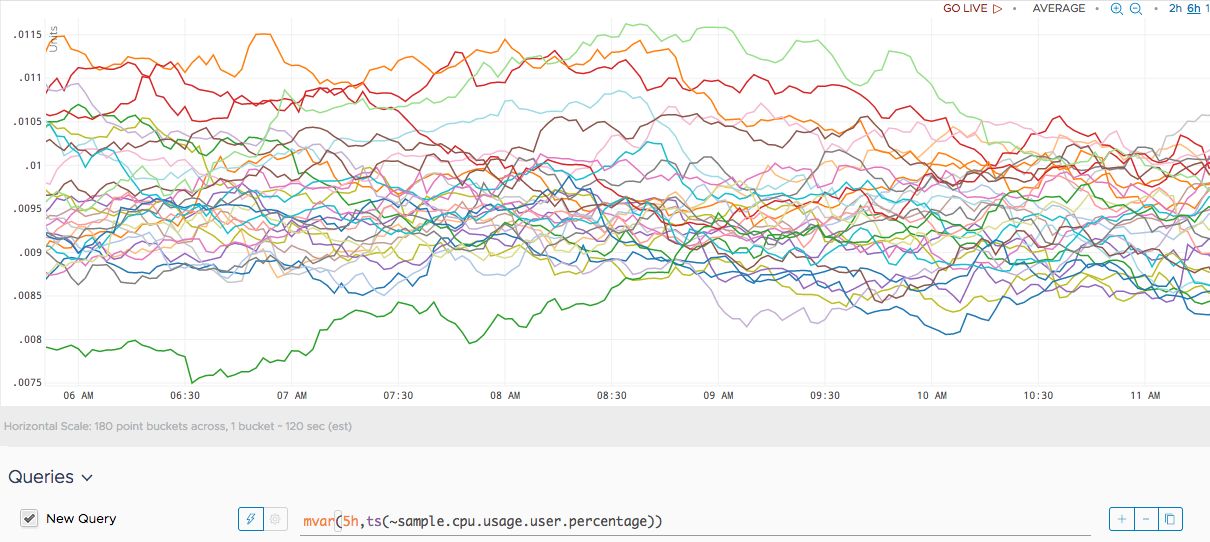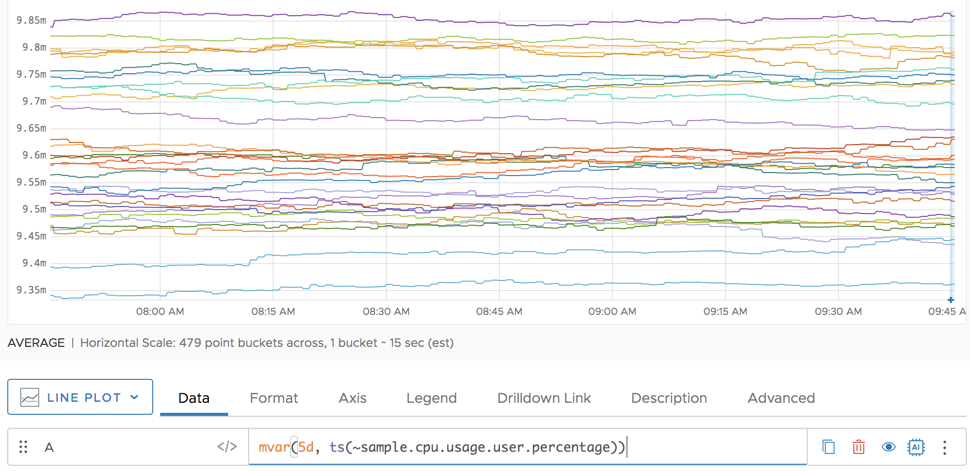Reference to the mvar() function

## Summary

``````mvar(<timeWindow>, <tsExpression>)
``````

Returns the moving variance for each series over the specified time window.

## Parameters

ParameterDescription
timeWindow Amount of time in the moving time window. You can specify a time measurement based on the clock or calendar (1s, 1m, 1h, 1d, 1w), the window length (1vw) of the chart, or the bucket size (1bw) of the chart. Default is minutes if the unit is not specified.
tsExpression Expression that describes the time series you want moving variances for.

## Description

The `mvar()` function computes the moving variance of each time series over a shifting time window. For example, `mvar(60m, ts(my.metric))` returns, at each point, the variance in the data values over the previous 60 minutes for each specified time series.

You can apply the `sqrt()` function to `mvar()` to get the moving standard deviation, for example: `sqrt(mvar(120m, ts(my.metric)))`.

## Example

The following example computes the moving variance for the CPU usage user percentage over 5 hours.We can see that the variance flattens out as we look at 5 days.